F Reviewing Complex DBA Setup

This appendix contains these topics:

The following examples show how to set up certain specific types of DBAs. These DBAs do not exist in the DEMO data.

This section contains the following examples:

• Example 1: Deduction Using Minimum Hours and Monthly Limits

• Example 2: Two Limits for 401k Deduction

• Example 3: Tax Exempt Deductions Section 125 and 401(k)

• Example 4: DBAs with Prior Limits

F.1 Example 1: Deduction Using Minimum Hours and Monthly Limits

This deduction is for a flat dollar amount, \$41.72, with \$ in the Method of Calculation. The deduction is based on a minimum of 40 hours worked per month using the Minimum Hours field. You will calculate deductions once a month, so set a monthly limit using a month-to-date Source of Calculation. This is flagged to calculate each pay period.

Figure F-1 DBA Setup screen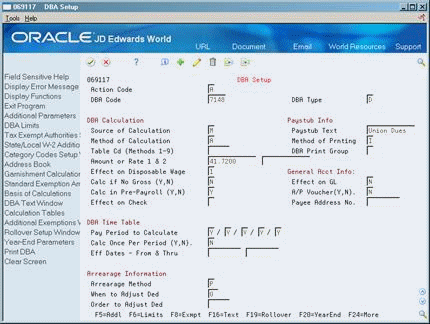Description of ''Figure F-1 DBA Setup screen''

If an employee did not work the required 40 hours in a pay period, no deduction exists. If the deduction was withheld the first pay period of the month, the system would not calculate the deduction in subsequent pay periods because of the monthly limit.

Figure F-2 DBA Limit window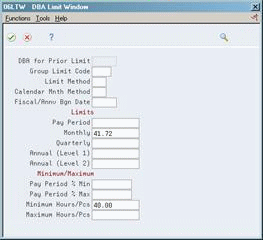Description of ''Figure F-2 DBA Limit window''

Figure F-3 DBA Additional Information window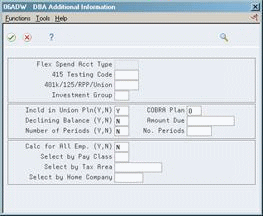Description of ''Figure F-3 DBA Additional Information window''

The DBA is flagged as included in a union plan for reporting purposes.

F.2 Example 2: Two Limits for 401k Deduction

This 401k deduction uses two annual limits, and therefore requires two DBAs. The deduction stops calculating when the year-to-date gross pay reaches \$150,000 or the year-to-date contribution reaches \$9,240.

Figure F-4 DBA Setup screen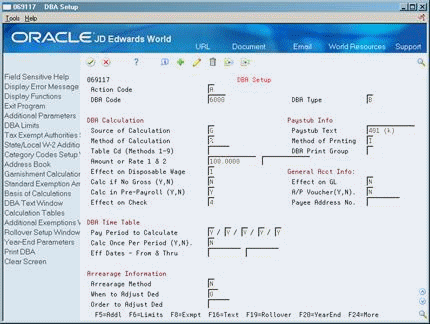Description of ''Figure F-4 DBA Setup screen''

The first DBA, 6000, tracks the year-to-date gross. It will stop calculating when the \$150,000 limit is reached.

Figure F-5 DBA Limit window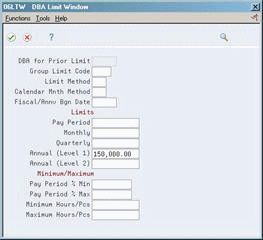Description of ''Figure F-5 DBA Limit window''

The second DBA, 6001, calculates the 15% deduction. It will stop calculating when the \$9,240 is reached.

Figure F-6 DBA Setup screen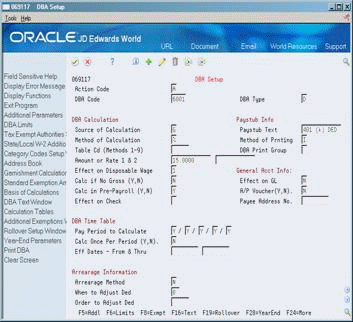Description of ''Figure F-6 DBA Setup screen''

Figure F-7 DBA Limit Window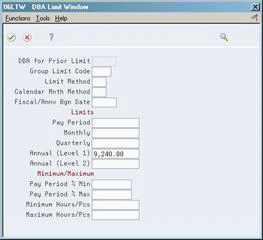Description of ''Figure F-7 DBA Limit Window''

It is based on DBA 6000. When DBA 6000 stops calculating, DBA 6001 will be based on zero amount and calculate zero for the deduction.

Figure F-8 Basis of Calculations screen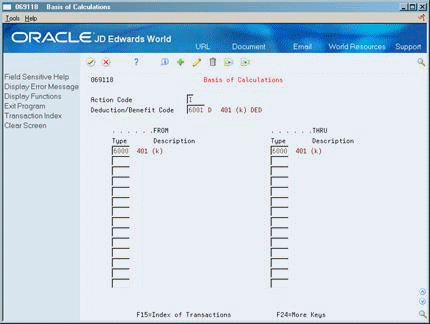Description of ''Figure F-8 Basis of Calculations screen''

The tax exempt status is not illustrated here.

F.3 Example 3: Tax Exempt Deductions Section 125 and 401(k)

You need the following DBAs:

• A tax exempt deduction to reduce taxable gross for Section 125. See example DBA 4227.

• A tax exempt deduction for 401k to calculate on the reduced taxable gross amount. See example DBA 7007.

• An intermediate benefit to hold the amount of the first deduction as a negative amount so that the system includes it in the basis for the second deduction. See example DBA 4228.

Figure F-9 DBA Setup screen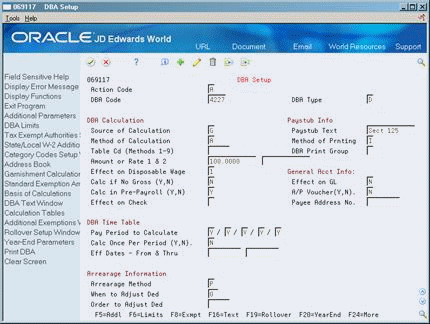Description of ''Figure F-9 DBA Setup screen''

This benefit holds the amount (\$100) as a negative amount to reduce taxable gross for the next pre-tax deduction. It will not print on the check, nor will it create a journal entry. Its basis of calculation is the Section 125 deduction only.

Figure F-10 DBA Setup screen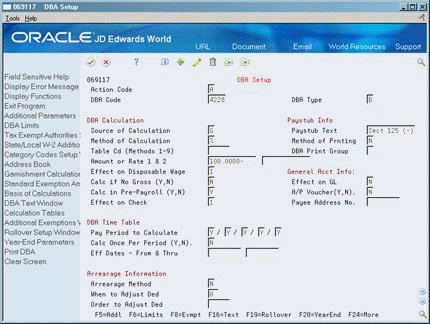Description of ''Figure F-10 DBA Setup screen''

Figure F-11 Basis of Calculations screen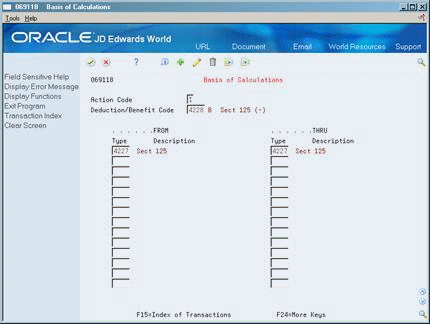Description of ''Figure F-11 Basis of Calculations screen''

The second deduction, the 401k, is set up as usual, with the basis of calculation including the negative amount of the Section 125 deduction. It is then based on the gross less the Section 125 deduction.

Figure F-12 DBA Setup screen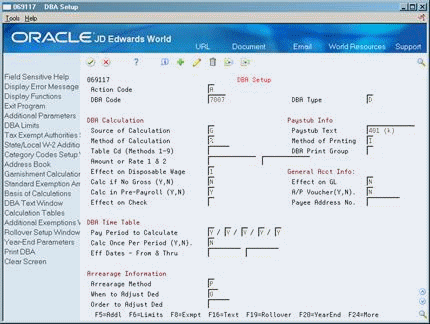Description of ''Figure F-12 DBA Setup screen''

Figure F-13 Basis of Calculations screen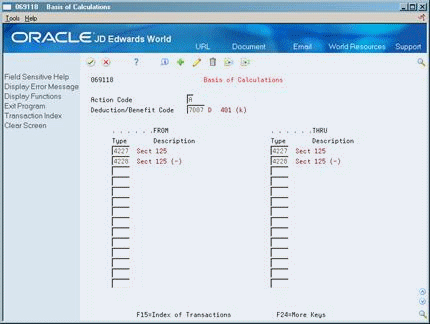Description of ''Figure F-13 Basis of Calculations screen''

The tax exempt status is not illustrated here.

F.4 Example 4: DBAs with Prior Limits

When you use DBA for Prior Limit, the system calculates the DBA with the higher number in the pay period after the first DBA reaches its limit.

The following sample shows a DBA with a higher number calculated in the same pay period that the first DBA reaches its limit. It requires setting up an intermediate DBA.

1. DBA 6670 calculates 3% of the gross salary up to an annual limit of 840.

Figure F-14 DBA Setup screen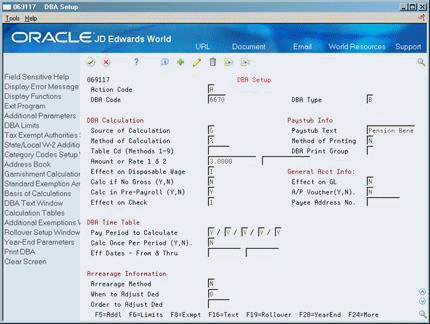Description of ''Figure F-14 DBA Setup screen''

Figure F-15 DBA Limit Window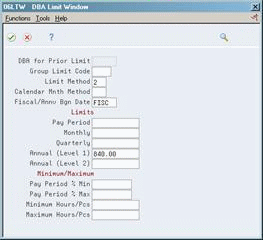Description of ''Figure F-15 DBA Limit Window''

2. DBA 6671 also calculates 3% of gross salary and stores it as a negative amount.

Figure F-16 DBA Setup screen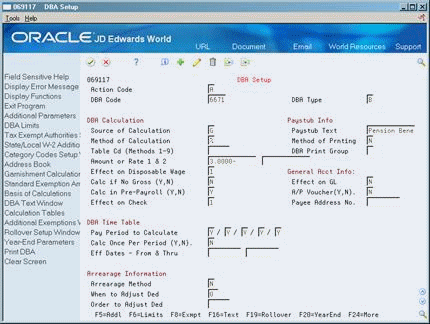Description of ''Figure F-16 DBA Setup screen''

There is no annual limit. When the system stops calculating DBA 6670, calculations continue for DBA 6671.

3. DBAs 6670 and 6671 combine to calculate DBA 6672.

Figure F-17 DBA Setup screen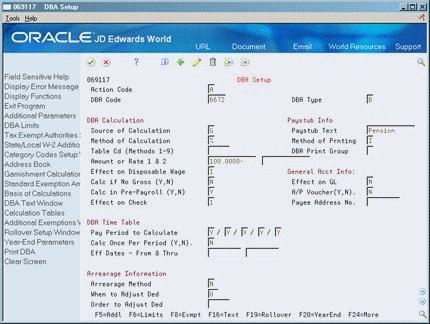Description of ''Figure F-17 DBA Setup screen''

Figure F-18 Basis of Calculations screen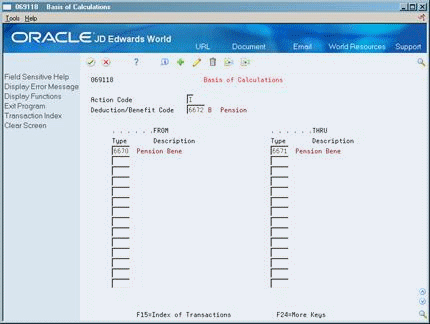Description of ''Figure F-18 Basis of Calculations screen''

While DBA 6670 continues to calculate, DBA 6670 + DBA 6671 = 0. When DBA 6670 reaches the annual limit and the system stops calculating it, DBA 6670 + DBA 6671 = positive 3% of gross.

You can change DBA Type, tax exempt status, method of calculation, and other values.